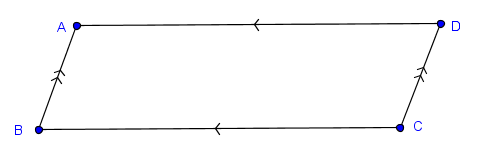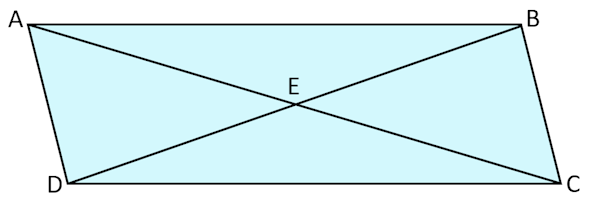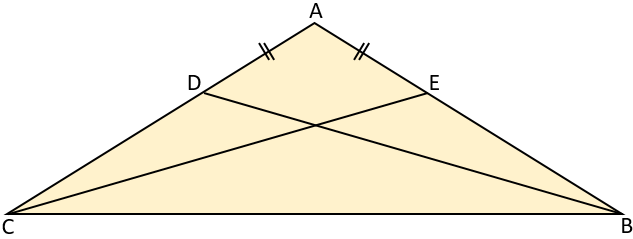# Exam-Style Questions.

## Problems adapted from questions set for previous Mathematics exams.

### 1.

GCSE Higher

Prove that triangle ABC is congruent to triangle CDA if ABCD is a parallelogram.### 2.

GCSE Higher

The design below is made from six congruent trapezia and two red triangles.

Each trapezium has one line of symmetry.1. The red triangles are isosceles triangles.
2. The red triangles are equilateral triangles.
3. The red triangles are congruent triangles.

(a) Which statements must be true?

(b) Which statements could be true?

(c) Which statements must be false?

### 3.

GCSE Higher

ABCD is a parallelogram with diagonals meeting at E. Prove that triangle ABE is congruent to triangle CDE.### 4.

GCSE Higher

ABC is an isosceles triangle in which AB = AC.

Prove that triangle ABD is congruent to triangle ACE.If you would like space on the right of the question to write out the solution try this Thinning Feature. It will collapse the text into the left half of your screen but large diagrams will remain unchanged.

The exam-style questions appearing on this site are based on those set in previous examinations (or sample assessment papers for future examinations) by the major examination boards. The wording, diagrams and figures used in these questions have been changed from the originals so that students can have fresh, relevant problem solving practice even if they have previously worked through the related exam paper.

The solutions to the questions on this website are only available to those who have a Transum Subscription.

Exam-Style Questions Main Page

Search for exam-style questions containing a particular word or phrase:

To search the entire Transum website use the search box in the grey area below.